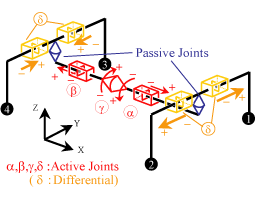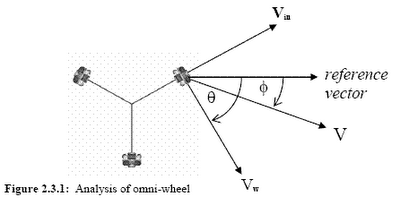## Thursday, August 03, 2006

### How to control omni-direction wheel

Omni-wheeled system motion
We now consider the motion of a simple omni-wheeled system,
where rotation is fixed. Consider Figure 2.3.1, showing the vectors
acting on one driving wheel.where, Vw is the velocity of the wheel, θ is the reference wheelangle, Vin is the induced velocity on wheel, φ is the reference body
velocity angle, and Vb is the body velocity of robot.
Now Vin and Vw are always orthogonal:

Vb2 = Vw2 + Vin2 (1)
Also:
Vin2 = Vb2 + Vw2 - 2 Vw Vb cos(θ - φ)
= Vb2 + Vw2 - 2 Vw Vb (cosθ cosφ + sinθ sinφ) (2)
Substituting (2) into (1), we may obtain:
Vw = Vb(cosθ cosφ + sinθ sinφ ) (3)For a given rotational velocity of the centre of mass, w, each
wheel must apply velocity:
Vw= Rw (4)
where R is the distance of the wheel from the centre of mass.
Thus, for each wheel:
Vw = Vb(cosθ cosφ + sinθ sinφ ) + Rw (5)
This is a general equation that is independent of the number
of wheels. Consider a three wheeled omni-directional vehicle
with wheels arranged at angles of 0°, 120° and 240°, equation (5)
yields:
Wheel 1 (θ = 0°): Vw 1 = Vb cosθ +Rw
Wheel 2 (θ = 120°): Vw 2= Vb (-0.5 cosθ+0.866 sinθ)+Rw
Wheel 3 (θ = 240°): Vw 3= Vb (-0.5 cosθ-0.866 sinθ)+Rw

from
Mark Ashmore and Nick Barnes, Omni-drive robot motion on curved
paths: The fastest path between two points is not a straight-line

Omni-wheeled